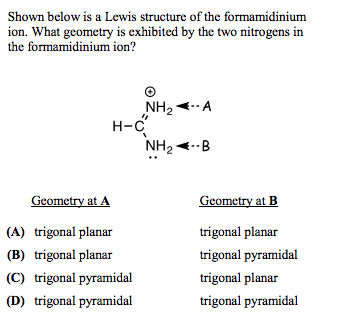Determine the geometry of each nitrogen atom in formamidinium ionThe geometry at A seems to be straightforward: $\ce{N}$ is surrounded by $\ce{C}$ and two $\ce{H}$'s. By VSEPR, this is trigonal planar.

For the geometry at B: $\ce{N}$ is surrounded by $\ce{C}$, two $\ce{H}$'s, as well as a lone pair. Once again by VSEPR, I determined this was trigonal pyramidal.

However, the answer was given as A, which means that my process for the geometry at B was incorrect. What mistake did I make and how should I fix it?

• Mar 3 '18 at 17:16

The hybridisation of any atom is determined by the total count of its sigma bonds and localised lone pairs. This is because, while localised lone pairs occupy hybrid orbitals (as in ammonia), delocalised lone pairs occupy pure $\ce{p}$ orbitals instead. Hence, the latter is not counted in determining hybridisation.

The $\ce{N}$ atom labelled "B" has its lone pair in conjugation with a pi bond. This is perfect for delocalisation/resonance to take place. Hence, that lone pair is not counted in determining hybridisation. Thus, it is also a trigonal planar geometry.

Interestingly, notice that the formamidinium ion has two equivalent canonical resonating structures. The lone pair of the atom B on delocalisation, results in a lone pair on A and a formal positive charge on B, which is just the reverse of the structure shown.

• Thank you for the prompt and clear answer! To clarify: Since it is a resonance structure in which the lone pair can either be on A or B, the lone pair is described as "delocalized," and can therefore be excluded from the VSEPR determination. Is that correct? Mar 3 '18 at 4:58
• @JohnLou It isn't necessary that the lone pair must be on A or B only. As long as the lone pair is delocalised, it is excluded from VSEPR determination. Mar 3 '18 at 5:00

This is a kind of a trick question (but it's tricking you to teach you an important point, so it's kind of justifiable).

The question says that the structure it shows is "a Lewis structure of the formamidinium ion," which is technically correct. But note that it doesn't describe it as the Lewis structure of the formamidinium ion, since the structure shown is really just one of the possible resonance structures of the molecule.

Indeed, it's easy to see that the arrangement of the atoms in the molecule is symmetric, and that the structure shown can be converted to its mirror image, with the nitrogens A and B swapped, just by shuffling electrons around. This symmetry strongly suggests that both the Lewis structure shown and its mirror image should be equally major contributors to the resonance, and that the actual minimum energy configuration of the molecule will be a symmetric hybrid of the two structures (and possibly other minor contributors), with both nitrogens having the exact same geometry and bonding.*

This symmetry argument rules out the asymmetric answers B and C, leaving only two plausible choices: either both nitrogens are trigonal planar, or they're both trigonal pyramidal. Since the Lewis structures contributing to the resonance feature a C=N double bond, their resonance hybrid must presumably involve some degree of π bonding between the central carbon and the nitrogens. This suggests that both nitrogens (and the central carbon) should be sp² hybridized, and thus planar.

*) In principle, the molecular geometry corresponding to the symmetric resonance hybrid structure could also be a local energy maximum, e.g. due to steric repulsion, with the true minimum energy geometry being asymmetric and (arbitrarily) favoring one of the mirror image Lewis structures over the other. Off the top of my head, I can't think of any specific example of a molecule where that happens, but I'm sure they must exist. For simple small molecules like this, though, it's usually a pretty safe bet that if a symmetric resonance hybrid can exist, it's probably the minimum energy configuration.